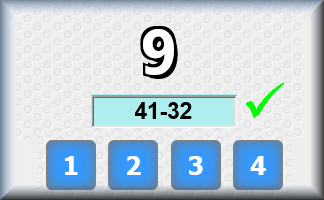## A Maths Lesson Starter of The Day# Four To Seven

Which of the numbers from one to twenty can you make with the digits:

4, 5, 6 and 7

You can use any mathematical operations but you must use all four digits once in each calculation.

Example 20 = 4(7 - 6) x 5

• Mr Kavanagh's Fifth Class, Cork, Ireland
•
• Our class figured out half of the solutions. We would have gotten more but we ran out of time.
• Alex Lee, NLCS Jeju
•
• Thease are the answers from my Y6 student.
1.(7-6)x(5-4)
2.(7-6)+(5-4)
3.(7-4)x(6-5)
4.(7-5)+(6-4)
5.square root(7-5)+(6-4)
6.4+(5+7)/6
7.56/4-7
8.(4+5)-(7-6)
9.(6+4)/5 +7
10.(4+7)-(6-5)
11.7x5-6x4
12.6x4-(5+7)
13.(6+7)x(5-4)
14.76/4-5
15.7^2-6x5-4
16.54/6+7
17.4x7-(5+6)
18.6x(5+7)/4
19.4x5-(7-6)
20.square root 4+6+5+7.
• Mrs Milne, Strandtown Primary School
•
• Our class really enjoyed this starter and as a class solved every one!

How did you use this starter? Can you suggest how teachers could present or develop this resource? Do you have any comments? It is always useful to receive feedback and helps make this free resource even more useful for Maths teachers anywhere in the world.

Previous Day | This starter is for 15 April | Next Day

### Here is a blank grid to collect in answers from the class

4, 5, 6 and 7

 1 = 2 = 3 = 4 = 5 = 6 = 7 = 8 = 9 = 10= 11 = 12 = 13 = 14 = 15 = 16 = 17 = 18 = 19 = 20 =

There are many different answers. These are not the only ones

 1 = (7 - 6)(5 - 4) 2 = 7 - 6 + 5 - 4 3 = 6(7 - 5) ÷ 4 4 = 7 + 6 - 5 - 4 5 = 5(√64 -7) 6 = √64 -7 + 5 7 = 64 - 57 8 = 6 - 7 + 5 + 4 9 = 74 - 65 10 = 7 - 6 + 5 + 4 11 = 75 - 64 12 = 7 + 6 - 5 + 4 13 =  67 - 54 14 = 7 + 6 + 5 - 4 15 = 5(6 + 4 - 7) 16 = 4(6 + 5 - 7) 17 = 5(6 - 4) + 7 18 = 74 - 56 19 = 5 x 4 - 7 + 6 20 = 4(7 - 6) x 5

## Change Numbers

Note to teacher: Doing this activity once with a class helps students develop strategies. It is only when they do this activity a second time that they will have the opportunity to practise those strategies. That is when the learning is consolidated. Click the button above to create another version of this Starter.

Your access to the majority of the Transum resources continues to be free but you can help support the continued growth of the website by doing your Amazon shopping using the links on this page. Below is an Amazon search box and some items I have chosen and recommend to get you started. As an Amazon Associate I earn a small amount from qualifying purchases which helps pay for the upkeep of this website.Teacher, do your students have access to computers?Do they have iPads or Laptops in Lessons? Whether your students each have a TabletPC, a Surface or a Mac, this activity lends itself to eLearning (Engaged Learning).Transum.org/go/?Start=April15

Here is the URL which will take them to a different type of mental maths activity.

Transum.org/go/?to=pairs21Here is the URL which will take them to a similar 4 digit calculation challenge.

Transum.org/go/?to=expressionFor All: